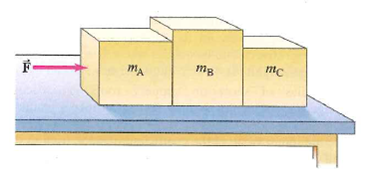# Problem: Three blocks on a frictionless horizontal surface are in contact with each other, as shown in Fig. 4–51. A force  is applied to block A (mass mA). (a) Draw a free-body diagram for each block. Determine (b) the acceleration of the system (in terms of mA, mB, and mC), (c) the net force on each block, and (d) the force of contact that each block exerts on its neighbor, (e) If mA = mB = mC = 12.0 kg and F = 96.0 N, give numerical answers to (b), (c), and (d). Do your answers make sense intuitively?

###### FREE Expert Solution

(a)

We'll represent the object with a dot.

We'll represent all the forces outward.

All the blocks have the weight acting downward.

All blocks have a normal force acting upward.

Block A:

Force F pushes to the right

Force FBA pushes to the left

80% (467 ratings)###### Problem Details

Three blocks on a frictionless horizontal surface are in contact with each other, as shown in Fig. 4–51. A force  is applied to block A (mass mA). (a) Draw a free-body diagram for each block. Determine (b) the acceleration of the system (in terms of mA, mB, and mC), (c) the net force on each block, and (d) the force of contact that each block exerts on its neighbor, (e) If mA = mB = mC = 12.0 kg and F = 96.0 N, give numerical answers to (b), (c), and (d). Do your answers make sense intuitively?